##### Pre-Calculus All-in-One For Dummies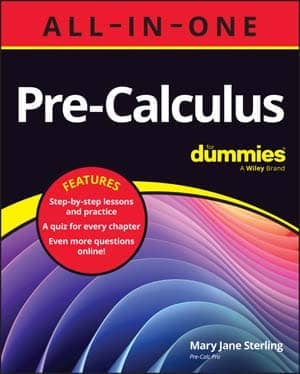One of the easiest tests you can use to help you decide whether a series converges or diverges is the nth term test. This test sort of looks at what's happening way out toward the "end" of an infinite list of numbers.

Of course, there isn't actually an end of an infinite list. You might say that the nth term test looks at what's happening to the numbers in the list the farther and farther you go out along the list.

Before looking at the formal definition of the nth test, you should be familiar with a sequence and a series.

A sequence is a finite or infinite list of numbers. When you add up the terms of a sequence, the sequence becomes a series. For example,

• 1, 2, 4, 8, 16, 32, 64,... is a sequence, and

• 1 + 2 + 4 + 8 + 16 + 32 + 64 + ... is the related series.

The nth term test: If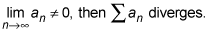In English, this says that if a series' underlying sequence does not converge to zero, then the series must diverge.

Of course, it does not follow that if a series' underlying sequence converges to zero, then the series will definitely converge. It may converge, but there's no guarantee.

Here's an example.

Question: Does the following converge or diverge?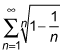You can answer this question with common sense if your calc teacher allows such a thing. As n gets larger and larger,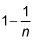increases and gets closer and closer to one. And when you take any root of a number like 0.9, the root is larger than the original number — and the higher the root index, the larger the answer is. So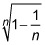has to get larger as n increases, and thus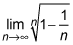cannot possibly equal zero. The series, therefore, diverges by the nth term test.

If your teacher doesn't like that approach, you can do the following: Plugging infinity into the limit produces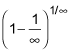which is 10, and that equals 1 — you're done. (Note that 10 is not one of the forms that gives you a L'Hôpital's Rule problem.) Because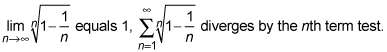(If your teacher is a real stickler for rigor, he or she might not like this approach either because, technically, you're not supposed to plug infinity into n even though it works just fine. Oh, well . . .)